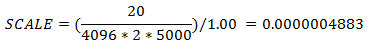﻿ Calculating SCALE values for EMG systems
 Required Parameters > The ANALOG group > ANALOG:SCALE

#### Calculating SCALE values for EMG systems

It is recommended that any device that has a user adjustable gain setting, as is typical with many EMG systems, should be scaled to deliver a signal in scaled in the output voltage range of the device.  This means that the C3D scale calculation, delivering a signal in terms of the device output voltage, does not have to be adjusted whenever the user changes the connected device gain.

However, if the system has a fixed gain, or a preset gain that will not be changed then data can be scaled accurately. For example, to use a case from the physical world, we will connect an external electromyography channel with a fixed gain of x5000 to the ADC system that we have previously described.  We will continue to use the same GEN_SCALE value of 1.00.  Using this 12-bit ADC (internal resolution of 4096) with range of ±10 volts and a gain of x2, produces an ANALOG:SCALE value of 0.0000004883Clearly, the individual ANALOG:SCALE values can become very small when the amplification factors are large – this is not always convenient, and under some circumstances can result in significant loss of precision.  For example, any application that only read the first six decimal places of the ANALOG:SCALE factor shown above would mistakenly determine the SCALE factor to be 0.000000 with the result that no analog data would be reported – review the analog scale calculations above for details.

In all of the examples used above, the ANALOG:GEN_SCALE parameter has been assigned a value of 1.00 – while this is convenient for the purposes of working these examples, this value is a factor in each of the individual ANALOG:SCALE calculations.  As a result, these values can be re-scaled by using a different GEN_SCALE value.

For instance, the first calculation above to scale the analog C3D data in volts measured at the ADC input used a GEN_SCALE value of 1.00 and produced a SCALE value of 0.004883.  If we recalculate the SCALE parameter using a GEN_SCALE value of 0.004883, we obtain an individual ANALOG:SCALE of 1.00 in that example and the prior calculation for an electromyography system now yields an ANALOG:SCALE value of 0.00010006.